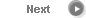#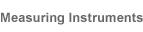# Precise Color Communication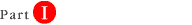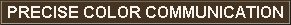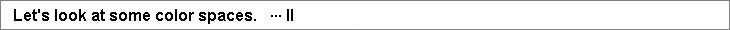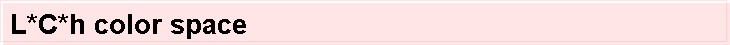The L*C*h color space uses the same diagram as the L*a*b* color space, but uses cylindrical coordinates instead of rectangular coordinates. In this color space, L* indicates lightness and is the same as the L* of the L*a*b* color space, C* is chroma, and h is the hue angle. The value of chroma C* is 0 at the center and increases according to the distance from the center. Hue angle h is defined as starting at the +a* axis and is expressed in degrees: 0 would be +a* (red), 90 would be +b* (yellow), 180 would be -a* (green), and 270 would be -b* (blue). If we measure the apple using the L*C*h color space, we get the results shown below. If we plot these values on Figure 9, we obtain point (A).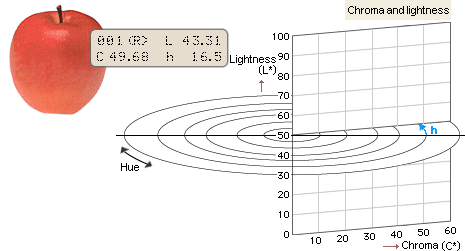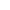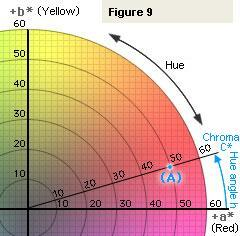Chroma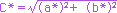Hue angle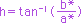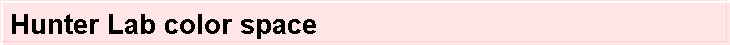The Hunter Lab color space was developed by R. S. Hunter as a more visually uniform color space than the CIE 1931 Yxy color space. Similar to the CIE L*a*b* space, it remains in use in various fields, including the paint industry of the US.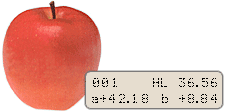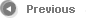8/13